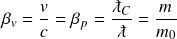# Velocity operator

The velocity operator quantifies a particle’s velocity, or rate of displacement. The operator is a value between 0 and 1 where 0 is the limit of minimum velocity and 1 is the speed of light.

The velocity operator uses a length dimension and a time dimension to quantify rates of change in a particle’s position, where position is not a point in space but a distribution over the particle’s wavelength. The maximum potential of the operator is equal to one unit of length per unit of time. The operator becomes smaller as units of length become smaller relative to units of time.

The New Foundations Model shows the velocity operator as a line across the unit dimensions. The potential in each unit dimension is reduced proportionally.

The velocity operator is the ratio of velocity to the maximum velocity potential, the speed of light

Velocity describes an intensive property of elementary particles in the rate of displacement. It can describe the displacement of a single particle or an entire system of particles.

A velocity may be represented using any distance or time value provided the ratio of the two is proportionally correct. Using a single particle’s wavelength as the change in distance gives the corresponding time of its oscillation period.The velocity operator transforms the maximum velocity potential into observable quantities of wavelength and oscillation period.

### Observable

The velocity operator quantifies the second part of the 2-part energy mechanism. For particles without rest mass, the operator is 1 and energy is maximized from this component. For particles with rest mass, the velocity operator reduces part II in the same proportion as Part I–the change in wavelength. This combination produces a squared quantity of kinetic energy relative to velocity.

For particles with rest mass, the velocity operator has an adjusted maximum potential at the Compton wavelength and not the Planck length. In non-relativistic terms, rest mass limits the particle’s potential for generating kinetic energy.

The following illustration shows the modified velocity scale. A particle’s maximum velocity potential corresponds with a particle’s maximum wavelength potential (its minimum wavelength), equal to the Compton wavelength. Rest mass is equal to its inertial mass at this limit.

## Velocity and momentum

For particles with rest mass, the velocity and momentum operators are equal, giving the same ratio of wavelength and velocity to their maximum potentials. The equivalence of these two operators is expressed in the ratiosThe momentum and velocity operators normalize unit dimensions of mass and momentum so that a measurement of a particle’s velocity also tells us the change in its wavelength and inertial mass. The two operators create a combined reduction of β2, or 𝑣2/𝑐2. This relationship is shown in the relational diagram of mechanical operators

Velocity operator